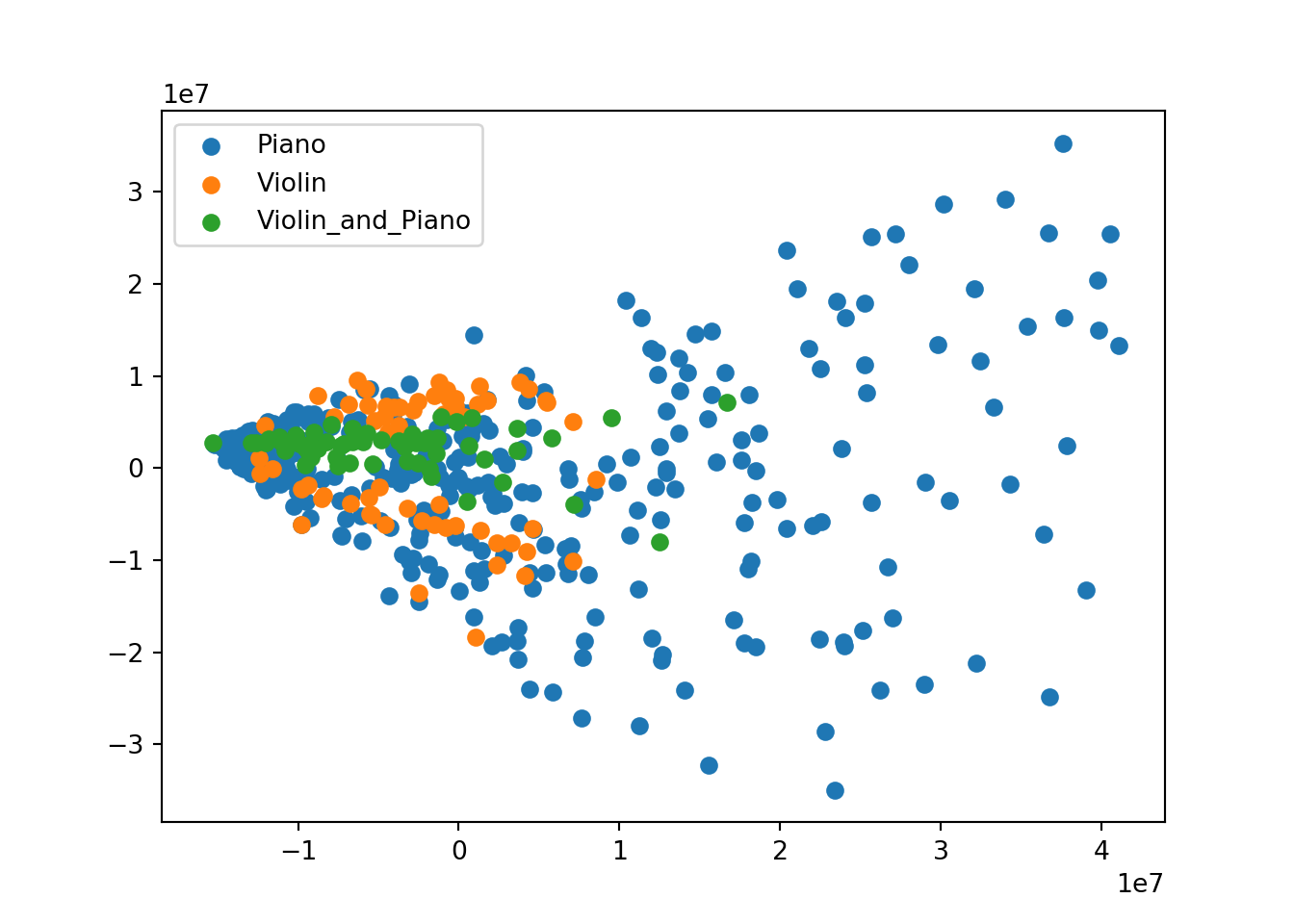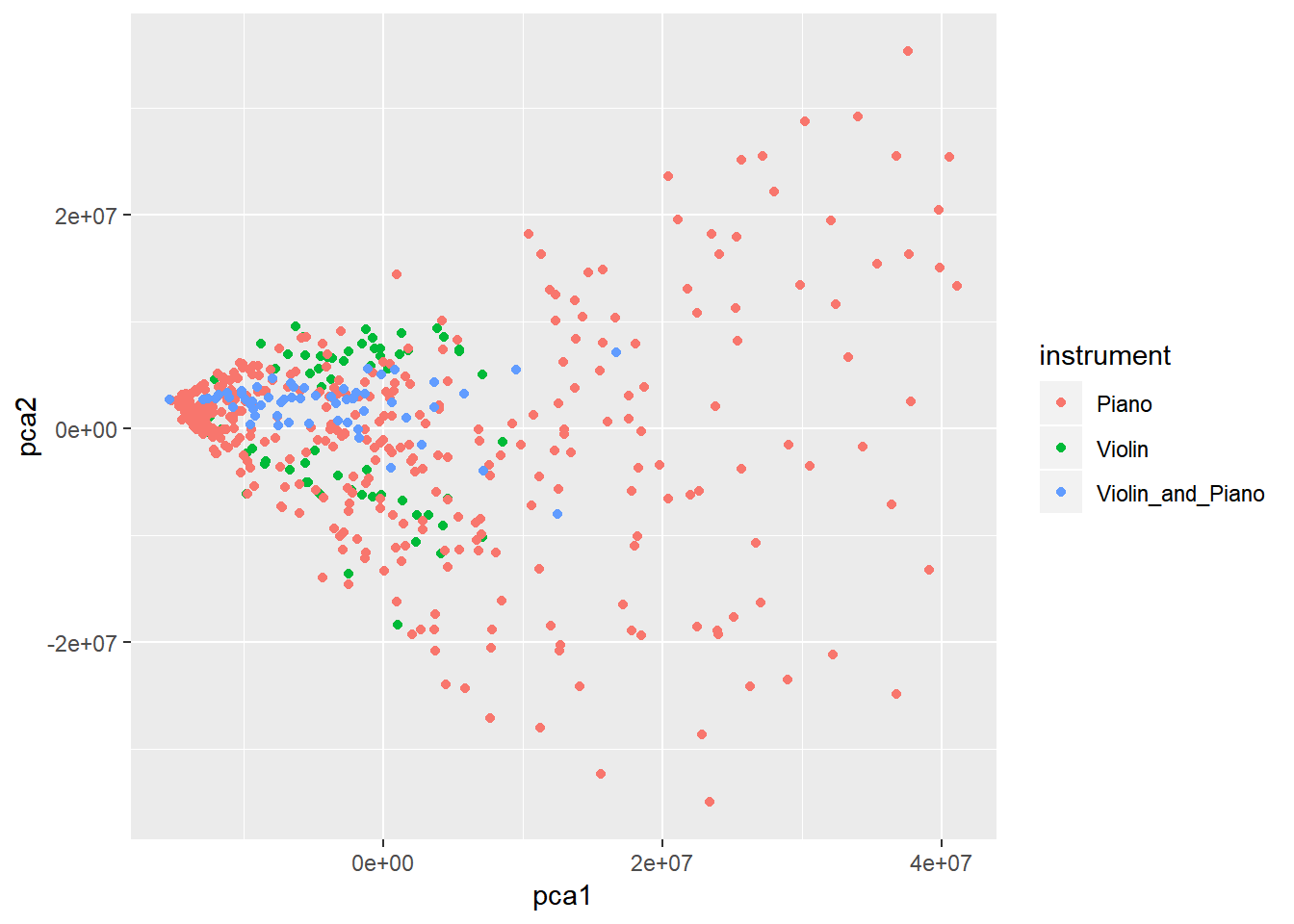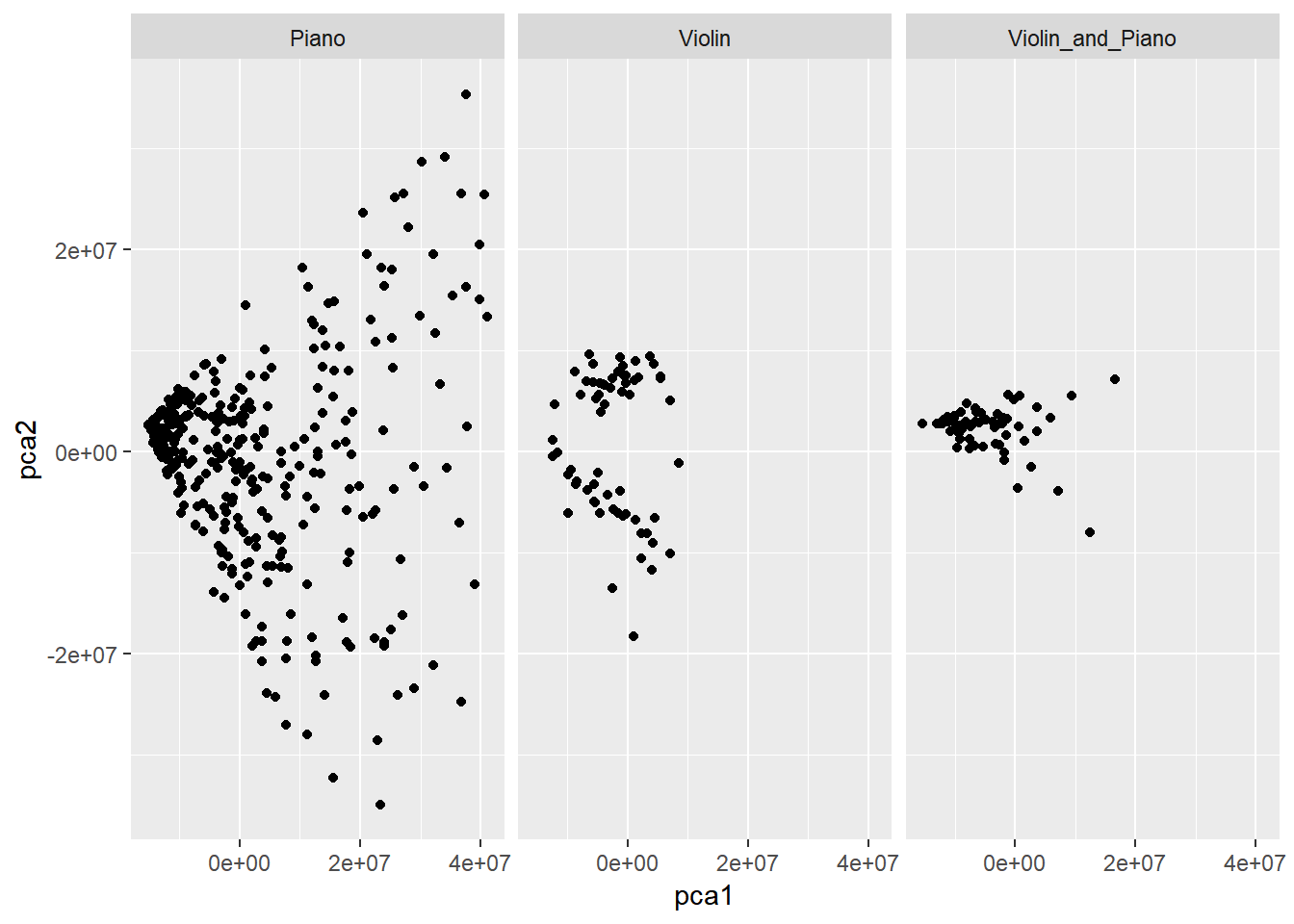# Introduction

You are going to write your own tutorial by combining your own notes and investigations with the code given in the slides. The first part of the tutorial aims to develop our baseline understanding of the link between the R and Python sessions and running code chunks interactively in R Markdown; the second part covers the importing, modelling and visualisation of the processed audio data.

The classification problem

We are going to work with some data derived from 9 pieces of music. Three examples are:

The example and the original Python code to process the audio files and carry out the machine learning analysis methods are by Michael Knight, University of Bristol.

Each of the pieces of music has been segmented into 5-second segments each of which has 5000 features. The features represent the apodised power spectrum of a 5-second segment.

We will try to classify these segments.

# Set up

## R packages needed

##########   R    ##########
library(reticulate)
library(ggplot2)
library(readxl)

## Python libraries needed

########## PYTHON ##########
import os
import pandas as pd

# for PCA
from sklearn.decomposition.pca import PCA

# for plotting
import matplotlib.pyplot as plt
# from mpl_toolkits.mplot3d import Axes3D

# Part 1: Building our understanding of the ‘reticulation’

This section is not required for the analysis of the data but is here to give us some practice in dealing with the linked sessions.

## Ways to find our working directory

A Python equivalent for R’s getwd() is from the os module.

########## PYTHON ##########
os.getcwd()
## 'C:\\Users\\Emma Rand\\Desktop\\useR2019\\useR2019_tutorial\\music_ml'

It prints out! with escaped ‘windows-way’ round slashes

Just like…

##########   R    ##########
getwd()
##  "C:/Users/Emma Rand/Desktop/useR2019/useR2019_tutorial/music_ml"

We can also use the the getcwd() method from os in a R chunk. Remember to access Python objects of any sort we use py$ Here we access the os object’s methods BUT in a R-like way using the $ not a .

##########   R    ##########
py$os$getcwd()
##  "C:\\Users\\Emma Rand\\Desktop\\useR2019\\useR2019_tutorial\\music_ml"

And access R functions in Python chunks in a Python-like way

########## PYTHON ##########
r.getwd()
## 'C:/Users/Emma Rand/Desktop/useR2019/useR2019_tutorial/music_ml'

## Ways to read in a file and calculate summary information

Let’s read one of the spectrum files in order to investigate passing dataframes between sessions. We can use the readxl1 package. I have added that to the R chunk named pkgs

##########   R    ##########
spectrum <- read_excel("Piano/01 Ballade No. 1, Op. 23_segments.xlsx")

How many segments (rows) are in this file?

##########   R    ##########
dim(spectrum)
##   100 5001

There are 100 segments. There are 5001 columns which are the segment label in the first column and the 5000 features. The features are named 0 to 4999

What is the mean and standard deviation of the second feature?

##########   R    ##########
mean_2 <- mean(spectrum$1) sd_2 <- sd(spectrum$1)

The second feature has a $$\bar{x} \pm s.d.$$ of 4422.34 $$\pm$$ 5711.78.

Access the dataframe in Python and use python to calculate those same values

Check it’s type:

########## PYTHON ##########
type(r.spectrum)
## <class 'pandas.core.frame.DataFrame'>
type(r.spectrum["1"])
## <class 'pandas.core.series.Series'>

The dimensions:

########## PYTHON ##########
r.spectrum.shape
## (100, 5001)

The mean and standard deviation:

########## PYTHON ##########
r.spectrum["1"].mean()
## 4422.34
r.spectrum["1"].std()
## 5711.784974979717

To read the file in in Python you can use the Pandas method pd.read_excel

########## PYTHON ##########
python_spectrum = pd.read_excel("Piano/01 Ballade No. 1, Op. 23_segments.xlsx")
########## PYTHON ##########
python_spectrum.shape
## (100, 5001)
##########   R    ##########
dim(py$python_spectrum) ##  100 5001 # Part 2: Classification of audio data ## The data Up to 100 segments were taken from each audio file although there are fewer for pieces shorter than 500 seconds. Instrument Piece Number of segments Piano 01 Ballade No. 1, Op. 23.m4a 100 Piano 1-01 Sonata No. 1 In F Sharp Minor, Op. 11_ I. Introduzione. un Poco Adagio ,Allegro Vivace.m4a 100 Piano 5-01 Beethoven_ Piano Sonata No. 14 in C-Sharp Minor, Op. 27 No. 2, ‘Moonlight’_ I. Adagio sostenuto.m4a 74 Piano 9-05 Beethoven_ Piano Sonata No. 29 in B-Flat Major, Op. 106, ‘Hammerklavier’_ I. Allegro.m4a 100 Violin 05 No.2 in A major RV 31_ I. Preludio a Capriccio. Presto.m4a 14 Violin 1-01 Sonata da chiesa a tre in F Major, Op. 1, No. 1_ I. Grave.m4a 15 Violin 1-02 Sonata da chiesa a tre in F Major, Op. 1, No. 1_ II. Allegro.m4a 18 Violin 24 No.11 in D major RV 9_ II. Fantasia. Presto.m4a 16 Violin and Piano 3-01 Sonata for Piano and Violin in F, K. 376_ I. Allegro.m4a 59 There are two xlsx files for each piece. • xxxxxx_segments.xlsx has the segments in rows and the features in columns • xxxxxx_SegmentInfo.xlsx has the metadata for each segment: the name of the piece, the instrument label on the piece, the start and end time of the segment. There are 496 segments in total of which 374 are from piano pieces and 63 are from violin pieces. The remaining 59 pieces are from Mozart’s Sonata piano and violin. ## Data import This will be carried out with Python. The nine "_segments.xlsx" files are read in to a single Pandas dataframe, df_seg. This requires nested for loops to iterate through the directories and through the files in the directories. The information about the segments in "_SegmentInfo.xlsx" files are similarly read into a Pandas dataframe, df_info and a column is added to capture the instrument labeling. ########## PYTHON ########## # read data in dirs_to_use = ["Violin", "Piano", "Violin_and_Piano"] df_seg = None df_info = None for d in dirs_to_use: for f in os.listdir(d): if f.endswith("segments.xlsx"): if df_seg is None: df_seg = pd.read_excel(os.path.join(d, f)) else: df_seg = df_seg.append(pd.read_excel(os.path.join(d, f)), ignore_index = True) elif f.endswith("SegmentInfo.xlsx"): if df_info is None: df_info = pd.read_excel(os.path.join(d, f)) df_info["Instrument"] = pd.Series([d] * len(df_info), index = df_info.index) else: df = pd.read_excel(os.path.join(d, f)) df["Instrument"] = pd.Series([d]*len(df), index = df.index) df_info = df_info.append(df, ignore_index = True)  ########## PYTHON ########## # check all is well type(df_seg) ## <class 'pandas.core.frame.DataFrame'> df_seg.shape ## (496, 5001) type(df_info) ## <class 'pandas.core.frame.DataFrame'> df_info.shape ## (496, 6) ## Analysis ### PCA in Python ########## PYTHON ########## # Apply PCA mdl = PCA() new_data = mdl.fit_transform(df_seg) type(new_data) ## <class 'numpy.ndarray'> access info from the new_data object ### Visualising the 🐍 PCA #### First using Python Biplot ########## PYTHON ########## # booleans for instrument p = df_info["Instrument"] == "Piano" v = df_info["Instrument"] == "Violin" pv = df_info["Instrument"] == "Violin_and_Piano" plt.figure() plt.scatter(new_data[p, 0], new_data[p, 1], label = "Piano") plt.scatter(new_data[v, 0], new_data[v, 1], label = "Violin") plt.scatter(new_data[pv, 0], new_data[pv, 1], label = "Violin_and_Piano") plt.legend() plt.show()#### Now using R Biplot ########## R ########## df <- data.frame(pca1 = py$new_data[ ,1],
pca2 = py$new_data[ ,2], instrument = py$df_info\$Instrument)

ggplot(data = df, aes(x = pca1, y = pca2, color = instrument)) +
geom_point()##########   R    ##########
ggplot(data = df, aes(x = pca1, y = pca2)) +
geom_point() +
facet_grid(.~instrument)